# User:Tohline/Appendix/Ramblings/RiemannB74C692

(Difference between revisions)
+ Figure 1a
+ [[File:EFE_STypeRiemannDiagram05.png|325px|EFE Parameter Space]] +
+   + + $~\frac{b}{a} = 0.41$ + +   + + Figure 1b
+ $~\frac{c}{a} = 0.385$ +
+ ''Direct'' + +   + + ''Adjoint'' +
+ $~\Omega_\mathrm{EFE} = 0.547874$ + + $~\Omega_\mathrm{EFE} = - 0.079886$ +
+ $~\lambda_\mathrm{EFE} = 0.079886$ + + $~\lambda_\mathrm{EFE} = - 0.547874$ +
+ + + + + + + + + + + + + + + + + + + + + + + + + + + + + The subscript "EFE" on Ω and λ means that the relevant frequency is given in units that have been adopted in [[[User:Tohline/Appendix/References#EFE|EFE]]], that is, in units of $~[\pi G\rho]^{1 / 2}$.  In Figure 1a, the solid purple circular marker (where the pair of purple lines cross) identifies the location of this model in the "c/a versus b/a" diagram that appears as Figure 2 on p. 902 of [http://adsabs.harvard.edu/abs/1965ApJ...142..890C S. Chandrasekhar (1965)]; essentially the same diagram appears in §49 (p. 147) of [[[User:Tohline/Appendix/References#EFE|EFE]]]. + =See Also= =See Also=

# Another S-type Example b74c692

This chapter is an extension of the chapter we have titled, "Riemann Meets COLLADA & Oculus Rift S." In that chapter we used as our first example of a Riemann S-type ellipsoid the model with parameters, b41c385. Other closely related chapters are listed below under the heading, "See Also".

## Key Physical Parameters

The model that we have chosen to use in our second successful construction of a COLLADA-based, 3D and interactive animation has the following properties; this model has been selected from Table 2 of our accompanying discussion of Riemann S-type ellipsoids: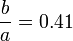$~\frac{b}{a} = 0.41$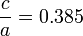$~\frac{c}{a} = 0.385$ Direct Adjoint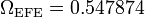$~\Omega_\mathrm{EFE} = 0.547874$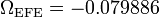$~\Omega_\mathrm{EFE} = - 0.079886$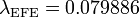$~\lambda_\mathrm{EFE} = 0.079886$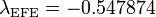$~\lambda_\mathrm{EFE} = - 0.547874$

The subscript "EFE" on Ω and λ means that the relevant frequency is given in units that have been adopted in [EFE], that is, in units of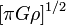$~[\pi G\rho]^{1 / 2}$. In Figure 1a, the solid purple circular marker (where the pair of purple lines cross) identifies the location of this model in the "c/a versus b/a" diagram that appears as Figure 2 on p. 902 of S. Chandrasekhar (1965); essentially the same diagram appears in §49 (p. 147) of [EFE].